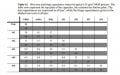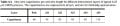# Capactiance of Metal Wire

#### thisonedude

Joined Apr 20, 2014
52
Problem A: An isolated metal4 wire is 1.5 mm long, 0.5 um wide, and runs over a large sheet of metal2. It is driven by a CMOS driver with very fast output rise and fall times. How will the delay change for the following cases? You may give your answer in a general sense that is true for all technologies, or use 0.25 um data from book pp. 143-145 or inside cover.
1. [4 pts] If the wire length is doubled.

So i'm confused about a small thing. The book gives an example about find the parallel plate and fringe capacitances, in that example, you have only Metal 1 and the substrate. In this problem, we are looking at metal 4 being over metal two. Do i also have to look at metal 2 when i'm calculating the fringe and parallel plate capacitance? The table gives both the top and bottom capacitor values. I took the values 6.5 and 14 for metal 4 and calculated the top plate. Do i also have to look at the metal 2 (Al2) coloumn to calculate the bottom plate. Or did i take the wrong vaues and was instead suppose to take the common capacitor value between Al4 and Al2 in order to get the proper capacitor value. Usin Al4 row values 6.5 and 14 i got: Cpp = 4.875fF and Cfringe=21fF. Am i approaching this problem right?#### thisonedude

Joined Apr 20, 2014
52
Just looking for direction/clarification. Don't want the answer just outright given.

#### WBahn

Joined Mar 31, 2012
28,163
The table gives the capacitance parameters BETWEEN the top plate and the bottom plate. So if you have a M4/M2 capacitor, then you use the M4 row and the M2 column to get the parameters you need.

You also have a capacitance between the large M2 sheet and whatever is under it, but that doesn't matter for the problem you are solving because it only asks about the capacitance seen by the M4 wire.

•thisonedude

#### thisonedude

Joined Apr 20, 2014
52
The table gives the capacitance parameters BETWEEN the top plate and the bottom plate. So if you have a M4/M2 capacitor, then you use the M4 row and the M2 column to get the parameters you need.

You also have a capacitance between the large M2 sheet and whatever is under it, but that doesn't matter for the problem you are solving because it only asks about the capacitance seen by the M4 wire.
Hi @WBahn I was wondering if you could check to see if i'm following the correct procedures for this problem. I think i confused myself as i was doing it.
First I calculated the unmodified time delay of the problem. From the table provided i took The value 15aF/um^2 for the area capacitance and 27af/um for the fringe capacitance. i then calculated the area of my m4 wire: LW = (1.5-10^3um)(.5um)=750um^2
So for my unmodified capacitances i calculated:
Cpp=(750um^2)(15aF/um^2)=11.25fF and for my fringe cap: Cfringe=(2)*(750um^2)*(27aF/um^2)=40.5fF.

Alright then i added them and got a total cap of 51.75fF. I plugged this into the time delay equation tp= ln(2)RC = R*(37.7075*10^-15).

For a i then calculated the new area, and new capacitances the same way:

Cpp=22.5fF, Cfringe=40.5fF, Ctot=63fF.

again i plugged into the time delay equation:
tp=(R)*(43.47*10^-15).

I assumed the resitnace for both the wires with different lengths were going to be the same and compared the value:

tpnew/tpold = 43.47/35.71 = 1.217. So the propagation delay will increase and the new elay would be 1.217 times higher than the old delay. Would this be a correct approach to the problem?

#### WBahn

Joined Mar 31, 2012
28,163
You are going to a lot of effort to answer a very simple question.

If you increase the length of the wire, will the capacitance go up or go down?

If the capacitance goes up, will the time delay go up or go down?

But if you are going to crank numbers, ask if the results make sense. If you double the length of the wire, wouldn't you expect the capacitance to double?

If the capacitance doubles, why would the time delay only go up by a factor of 1.217?

•thisonedude

#### thisonedude

Joined Apr 20, 2014
52
You are going to a lot of effort to answer a very simple question.

If you increase the length of the wire, will the capacitance go up or go down?

If the capacitance goes up, will the time delay go up or go down?

But if you are going to crank numbers, ask if the results make sense. If you double the length of the wire, wouldn't you expect the capacitance to double?

If the capacitance doubles, why would the time delay only go up by a factor of 1.217?
I see my mistake. yes if length goes up then cap goes up which means the delay will go up too. the delay will double since the length

#### WBahn

Joined Mar 31, 2012
28,163
I see my mistake. yes if length goes up then cap goes up which means the delay will go up too. the delay will double since the length
It may go up more than that because the resistance of the wire will double, too. But that may or may not be the dominant resistance (just like the wire capacitance may not be the dominant capacitance).